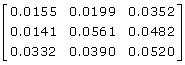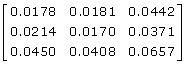# Table 6 Epistatic model - dominant (EPI DD).

Low risk

High risk

r1 = 2; r2 = 4

r1 = 5; r2 = 10

Theoretical penetrance matrixNeural network

Mean absolute difference ESum

0.3095

0.2524

Logistic regression

Mean absolute difference ESum

0.3132

0.6528

Logistic regression (design variables)

Mean absolute difference ESum

0.3071

0.2648

1. Mean absolute differences between theoretical and estimated penetrance matrices from 100 replications in the low and high risk scenario.